# 目标检测算法(1)目标检测中的问题描述和R-CNN算法

1. 目标检测中的一些基本概念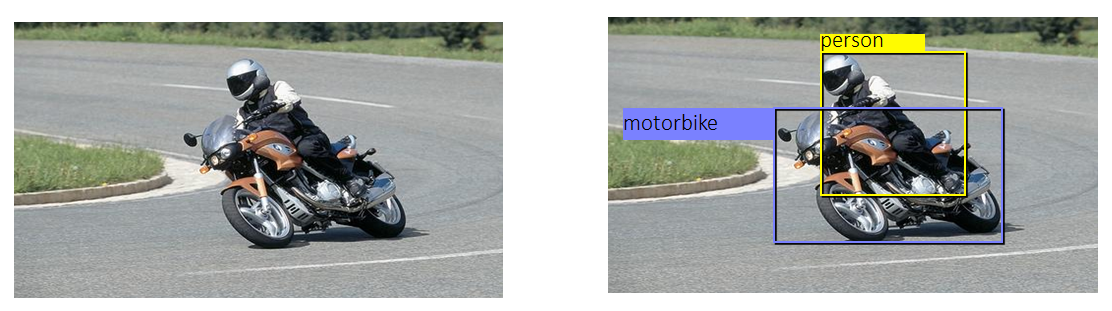图2 目标检测示例：左图是输入，右图是输出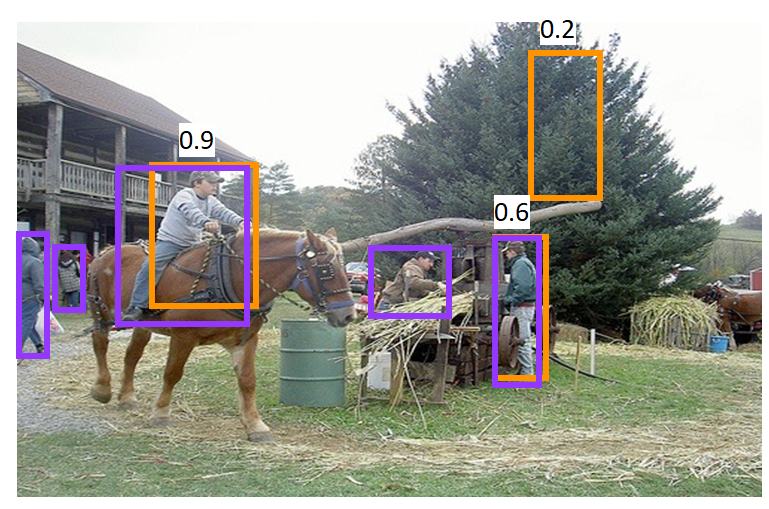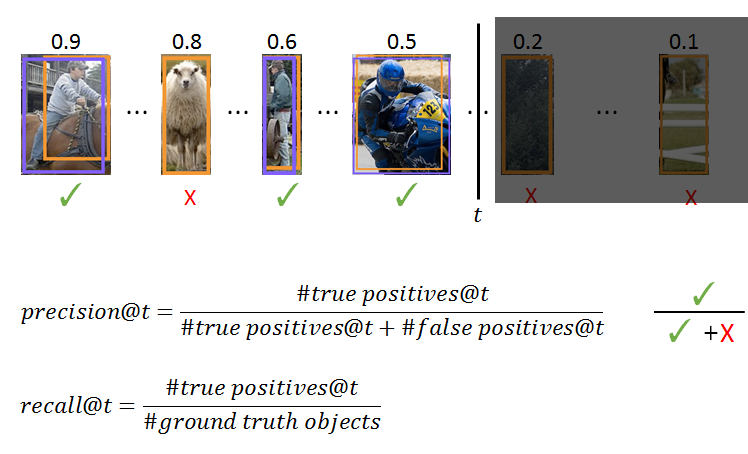$precision=\frac{true positive}{true positive+false positive}$

$recall=\frac{true positive}{ground truth}$

(1) true positive表示正确的预测为正类，在目标检测中就是指预测的bounding box和ground truth的IoU超过0.5的box的个数；

(2) false positive表示错误的预测为正类，对应于bounding box和ground truth的IoU小于等于0.5的box的个数，还有那些重复预测同一个ground truth的box的个数。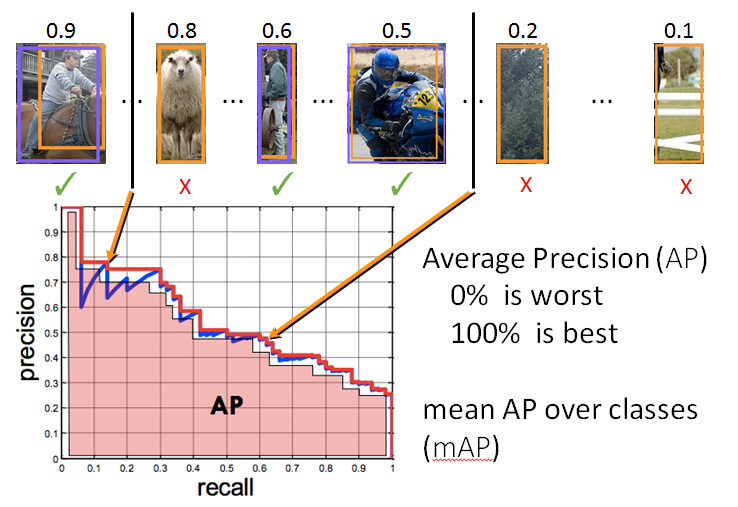图5 mAP计算示意图

(1) 怎样找出这些预测的bounding box？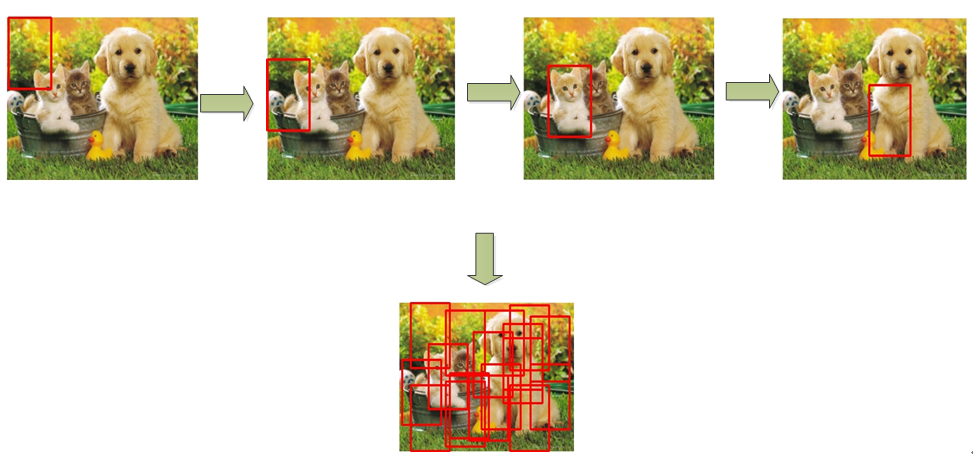(2) 假如我们已经找到了一个bounding box，怎样判断属于哪一个类？

(3) 是否需要对找到的包含物体的box进行修正？

带着这三个问题，这篇文章主要介绍目标检测领域一个里程碑的算法RCNN，即基于区域的卷积神经网络方法。

R-CNN（region with CNN feature）于13年被Ross Girshick（江湖人称RBG大神）提出，是使用深度学习进行目标检测的里程碑式的工作，RBG主页：http://www.rossgirshick.info，主页中列出的关于目标检测及相关领域的工作都非常经典，可以说这些工作很大程度推动了深度学习在目标检测中的应用。废话不多说，首先我们看看RCNN算法的动机。

1. 动机及解决的问题

(1) 与分类任务不同，目标检测需要框出指定的区域，有相关paper直接使用回归的方法得到区域的位置，但实际应用起来效果并不好；另外，有方法直接使用滑窗加深度学习的方法，不过这一类方法通常网络层次并不深，主要考虑到的是一旦网络层次加深，最后输出的特征图对应的原始图像的感受野太大，造成检测精度不高；

(2) 一个更好地解决方案是是使用所谓“区域建议(region proposal)”的方法给出一堆候选区域，这些候选区域能够先过滤掉那些大概率不包含物体的区域，然后选择一个深度神经网络抽取特征；

(3) 一旦抽取特征之后可以设计一个SVM分类器，即对每一个类别的物体都看成是一个单独的二元分类问题；

(4) 最后使用回归的方法对包含物体的bounding box进行回归，回归的目的是修正bounding box的偏差。

2. 算法架构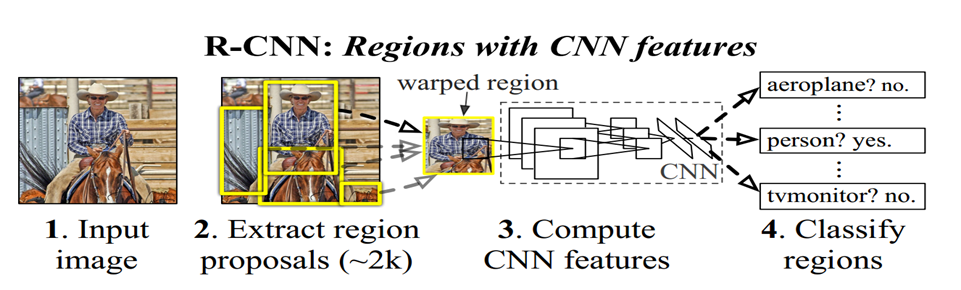RCNN的算法框架如图7所示，算法过程描述如下：

(1) 第一步，即图7中的1~2，对于给定的输入图像，使用选择性搜索(selective search)的区域建议方法提取出大约2000个候选区域，即首先过滤掉那些大概率不包含物体的区域，通过这一阶段将原始图像中需要处理的区域大大减少；

(2) 第二步，分为两个小步：

• 第一小步，需要对第一阶段中抽取得到的候选区域，经过一个叫做"warp"的过程，这个warp实际就是一个缩放的过程，因为第一步我们提取出的候选区域大小不一，但是后续接入的深度网络的输入是固定的，因此这些区域的大小需要适配CNN网络固定大小的输入；
• 第二小步，把第一小步中warp之后的候选区域接入到卷积神经网络，抽取一个相对低维的特征；

(3) 第三步，对应图中的3~4，对每个类都训练一个二元的线性SVM，然后判断上一步抽取的特征是否包含物体。

(4) 第四步，这一步图上并没有画出，这一步用于对包含物体的候选区域位置进行回归，以修正偏差。

3. 算法具体流程

(1) 怎样使用selective search方法提取候选区域

RCNN一大创新点是对原始图像抽取一些候选区域，再使用深度学习进行特征抽取，文中列举了一些区域建议的方法，最后使用的是selective search的方法：“Selective Search for Object Recognition”。selective search是一种“hierarchical bottom-up grouping”的方法，即层次、自底向上的分组方法，简单来说就是先找出图像中的相邻区域，然后定义一个领域相识度的计算规则，使用这些规则一直合并相似度较高的区域并且更新，最后知道覆盖至整张图像，完成处理的图像中不同的区域就是这些“region proposal”。以下是示意图：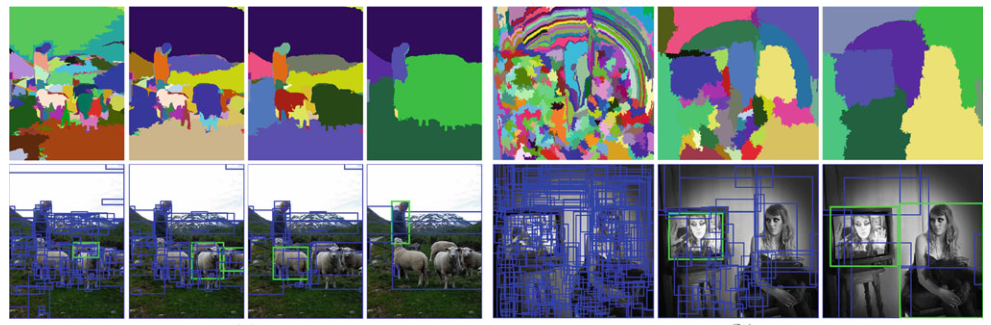a. 对于所有的相邻区域$(r_{i},r_{j})$，按照相似度规则计算相似度$s(r_{i},r_{j})$，并且计算$S=S\cup s(r_{i},r_{j})$，即计算所有领域的相似度集合；

b. 假如$S\neq \varnothing$：

• 获取$S$中相似度最高的一对区域$s(r_{i},s_{j})=max(S)$；
• 对最相似的两个区域进行合并$r_{t}=r_{i}\cup r_{j}$；
• 从$S$集合中去除和$r_{i}$及$r_{j}$相邻的相似区域；
• 计算合并后的区域和其邻域的相似度集合$S=S\cup S_{t}$及$R=R\cup R_{t}$
• 重复以上步骤直至$S= \varnothing$

c. 从集合$R$中提取出所有的boxes，即定位的矩形区域

$s(r_{i},r_{j})=a_{1}s_{colour}(r_{i},r_{j})+a_{2}s_{texture}(r_{i},r_{j})+a_{3}s_{size}(r_{i},r_{j})+a_{4}s_{fill}(r_{i},r_{j})$    $a\in \left \{ 0,1 \right \}$

(2) CNN网络输入

a. 各向异性（anisotropically）缩放，即对候选区域缩放的高和宽的倍数不同，直接拉成CNN中输入大小的形式，这种变换在文中称为“warp”；

b. 各向同性（isotropically）缩放，即缩放的高和宽的比例倍数相同，这里又分为两种：一种对原始的图像按等比例缩放后的灰色区域进行填充，另一种不进行填充。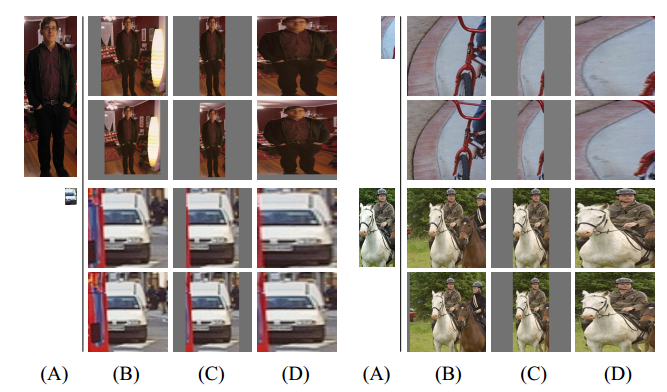图9 几种候选区域缩放的方法对比

(3) CNN网络训练

(4) SVM分类器的训练

(5) 非极大值抑制过滤重叠的bounding box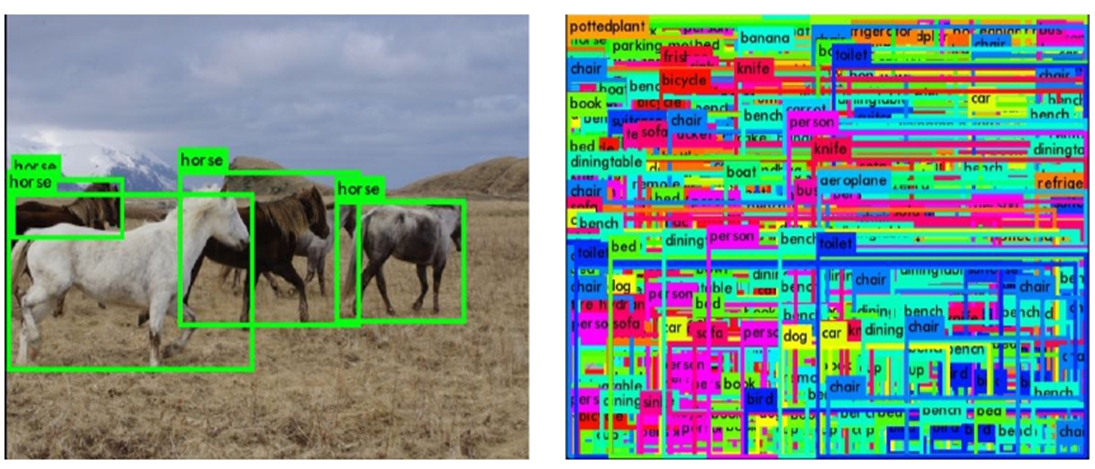(6) 检测时间分析

(7) 回归器修正box偏差

$\hat{G}_{x}=P_{w}d_{x}(P)+P_{x}$

$\hat{G}_{y}=P_{h}d_{y}(P)+P_{y}$

$\hat{G}_{w}=P_{w}exp(d_{w}(P))$

$\hat{G}_{h}=P_{h}exp(d_{h}(P))$

$w_{*}=\underset{\hat{w}_{*}}{argmin}\sum_{i}^{N}(t_{*}^{i}-\hat{w}_{*}\varnothing(P^{i}) )+\lambda \left \| \hat{w}_{*} \right \|^{2}$

$t_{x}=(G_{x}-P_{x})/P_{w}$

$t_{y}=(G_{y}-P_{y})/P_{h}$

$t_{w}=log(G_{w}/P_{w})$

$t_{h}=log(G_{h}/P_{h})$

(8) 为什么RCNN使用SVM替代softmax层进行分类？

1. 在Pascal VOC10-12以及ILSVRC2013 detection 的实验结果

2. 特征学习可视化

3. Ablation studies
Ablation studies 可以看成是去除算法中的某些结构，然后进行实验并比较分析实验的对比效果，比较了没有fine-tuning和有fine-tuning的效果对比；同时还分析了RCNN和两种不同的DPM方法（HOG+DPM和稀疏编码直方图+DPM，简称HSC+DPM），得出的结论是RCNN的性能也要好于二者。

4. 关于网络结构

5. 关于误差分析

RCNN是使用深度学习进行目标检测中非常有意义的一项工作，它首先利用选择性搜索选取一些区域候选框，然后通过CNN抽取特征并使用SVM进行分类，然后使用NMS排除重叠的bounding box，最后使用回归器对box的位置进行修正。一方面，RCNN在精度上和速度上相比较传统方法均有很大的提升，是一个有里程碑意义的研究；另一方面我们应该也可以看到，RCNN这篇论文中的工作量非常大，了解其中的细节便于我们理解目标识别领域的关键问题，同时RCNN也结合或借鉴了之前的算法，比如选择性搜索进行区域候选、使用NMS筛选窗口、DPM中的回归修正的思想等等，可以说是“站在前人的肩膀上”。通过分析我们可以得出RCNN的一些缺点：

(1) RCNN的耗时体现在两个阶段，一个是区域候选需要一定时间，另一个是对于每一个区域候选框都需要重新丢进网络里才能抽取特征，特别是后者导致RCNN非常的慢；

(2) RCNN在网络的输入是使用的warp方法，这种缩放会不会对最终的精度有影响？

(3) RCNN显然并不是一个端到端的工作，通常情况下我们认为端到端的工作可以达到更好的效果，因为不同模块之间的衔接可能会存在一定的信息损失。

posted @ 2018-08-24 10:26  supersayajin  阅读(6615)  评论(0编辑  收藏